# Harmonically Excited Support Motion of a Single DOF System

4.3 Differential equation for Support Motion

In many cases, the dynamical system is excited by the motion of the support point, as shown in Fig. 4.1. Let y be the harmonic displacement of the support point and x is the displacement of the mass m from the inertial reference as shown in Fig. 4.1.

###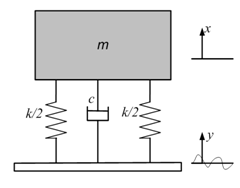Fig. 4.1: System excited by motion of support point

In the displaced position, the unbalance forces are due to the damper and the springs. So the differential equation of motion becomes,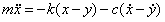(4.1)

Making the substitution          z = x – y

Equation (4.1) becomes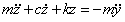(4.2)

Suppose y = Y sinωt has been assumed for the motion of the base. The solution can be written as,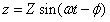(4.3)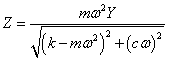(4.4)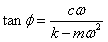(4.5)

After solving, the steady-state amplitude and phase can be written as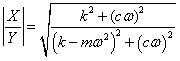(4.6)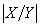is the amplitude of the absolute displacement of the mass to the amplitude of displacement of the base.

And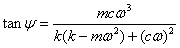(4.7)

The simulated curve for steady state amplitude and phase ψ against frequency ratio ω/ω nf  for a particular damping ratio of ζ = 0.190909 is shown in Fig. 4.2. Eq. (4.6)  & Eq. (4.7) were used to obtain the response for variation of amplitude    and phase ψ against frequency ratio ω/ω nf   at different damping ratios. From the simulated curve, operating the VI panel at different damping ratios, one can observe that the amplitude ratio is near to unity for small value of ω/ω nf . The absolute amplitude ratio has a value of one for ω/ω nf = √2. For (ω/ω nf )>√2, the amplitude ratio is less than one for all values of ζ.

###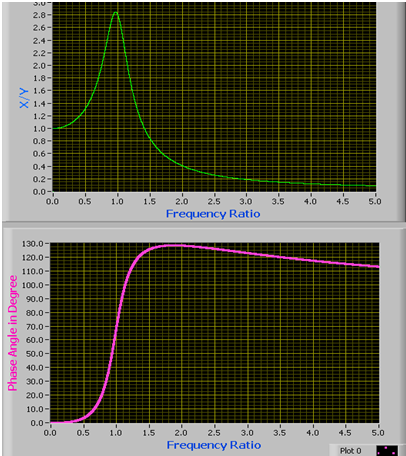Fig. 4.2: Response for support motion

Following points can be concluded from the simulated response

1.  The amplitude ratio is less than one for all values of damping when frequency ratio is greater than √2.

2.  For high values of frequency ratio (frequency ratio>> √2), the amplitude ratio is quite small which means that the support displacement is almost negligible.

3.  For high values of frequency ratio the damping does not have any significant role so far as the amplitude ratio is concerned.

4.  The phase angle is not 90 0 at resonance as in case of force excitation.